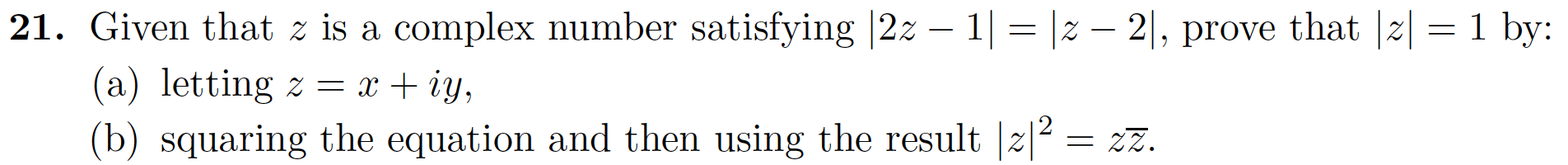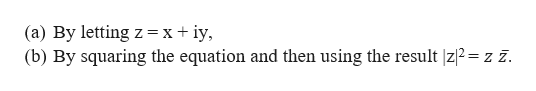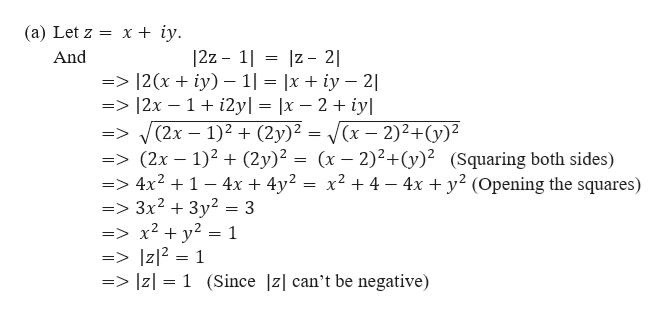# 21. Given that z is a complex number satisfying |2z 1 |z - 2|, prove that |z|(a) letting z = x + iy,(b) squaring the equation and then using the result z21 by:= 2z.

Question
1 views

Hi, there are two questions a) and b) attached

Thanks so Much!!!!help_outlineImage Transcriptionclose21. Given that z is a complex number satisfying |2z 1 |z - 2|, prove that |z| (a) letting z = x + iy, (b) squaring the equation and then using the result z2 1 by: = 2z. fullscreen
check_circle

Step 1

Given that z is a complex number satisfying |2z – 1| = |z – 2|. We need to prove that |z| = 1 by two different methods.help_outlineImage Transcriptionclose(a) By letting z= x + iy (b) By squaring the equation and then using the result |z|2=z z. fullscreen
Step 2

Now,

...help_outlineImage Transcriptionclose(a) Let z x + iy. 12z - 1 z 2| And => |2(x + iy) -1 = |x iy 2| => |2x - 1 i2yl = |x - 2 + iy => (2x- 1)2 + (2y)2 = (x- 2)2+(y)2 => (2x 1)2 (2y)2 => 4x21- 4x + 4y2 =>3x2 3y2 = 3 => x2 + y2 = 1 => |z12 = 1 => |z= 1 (Since |z| can't be negative) (x- 2)2+(y)2 (Squaring both sides) x2 4 4x2 (Opening the squares) fullscreen

### Want to see the full answer?

See Solution

#### Want to see this answer and more?

Solutions are written by subject experts who are available 24/7. Questions are typically answered within 1 hour.*

See Solution
*Response times may vary by subject and question.
Tagged in

### Math